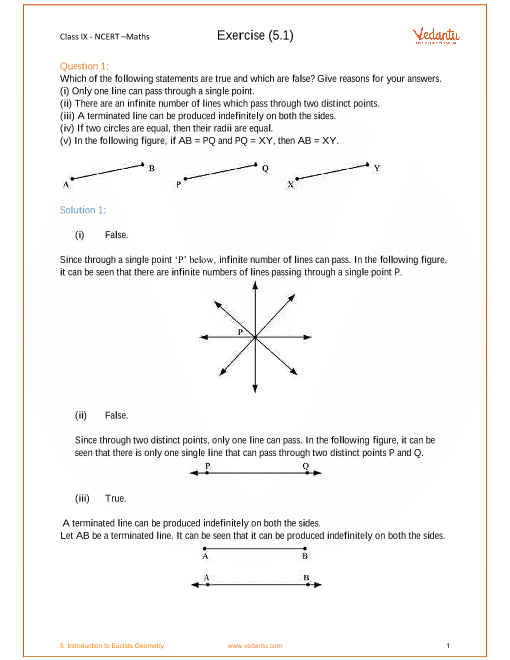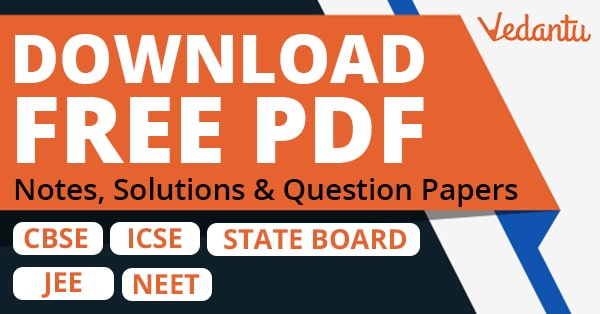NCERT Solutions for Class 9 Maths Chapter 5 Introduction To Euclid's Geometry (Ex 5.2) Exercise 5.2

NCERT Solutions for Class 9 Maths Chapter 5 Introduction To Euclid's Geometry (Ex 5.2) Exercise 5.2

Free PDF download of NCERT Solutions for Class 9 Maths Chapter 5 Exercise 5.2 (Ex 5.2) and all chapter exercises at one place prepared by expert teacher as per NCERT (CBSE) books guidelines. Class 9 Maths Chapter 5 Introduction To Euclid's Geometry Exercise 5.2 Questions with Solutions to help you to revise complete Syllabus and Score More marks. Register and get all exercise solutions in your emails.